# Use the graph to answer the following questions: most volatile: choose one Which liquid is the...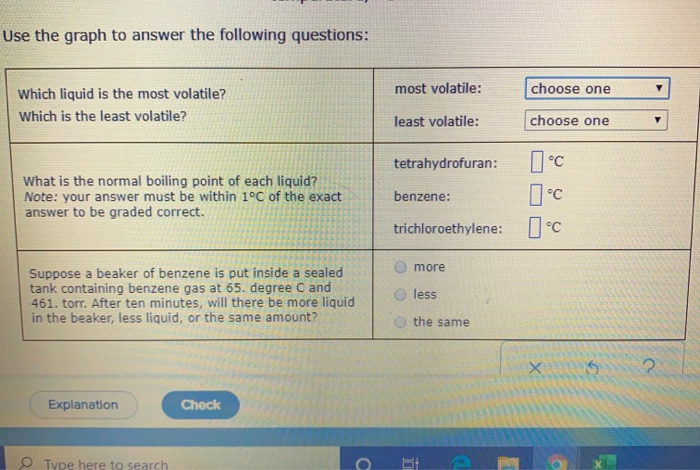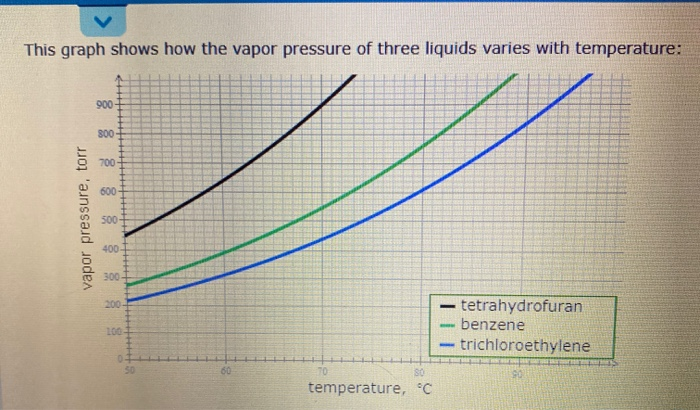Use the graph to answer the following questions: most volatile: choose one Which liquid is the most volatile? Which is the least volatile? least volatile: choose one tetrahydrofuran: 1 °C What is the normal boiling point of each liquid? Note: your answer must be within 1°C of the exact answer to be graded correct. benzene: trichloroethylene: 1 °C more Suppose a beaker of benzene is put inside a sealed tank containing benzene gas at 65. degree C and 461. torr. After ten minutes, will there be more liquid in the beaker, less liquid, or the same amount? less the same Explanation Check Tupe here to search O BE
This graph shows how the vapor pressure of three liquids varies with temperature: vapor pressure, torr tetrahydrofuran - benzene - trichloroethylene temperature, °C

Boiling point is the temperature at which vapour pressure of liquid become equal to the atmospheric pressure i.e 760mmhg or 760 torr

Hence from the graphn we can make following observations

Tetrahydrofuran ist most volatile and trichloroethylene is the least volatile liquid

Boiling point of benzene 79.9°c

Boiling point of Tetrahydrofuran 65°c

Boiling point of trichloroethylene 87°

#### Earn Coin

Coins can be redeemed for fabulous gifts.

Similar Homework Help Questions
• ### Use the graph to answer the following questions: most volatile: choose one Which liquid is the...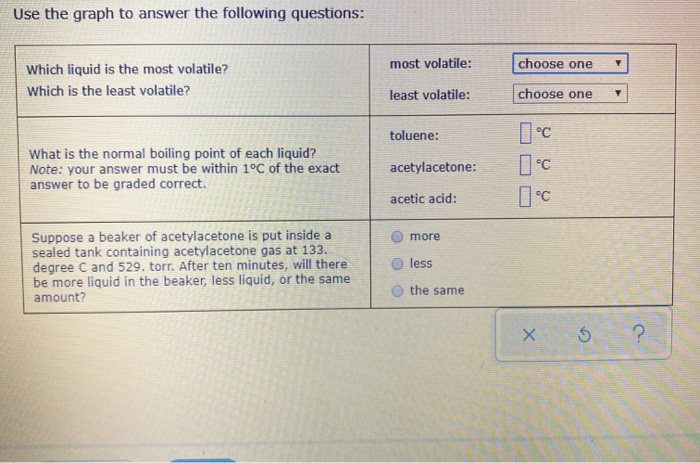Use the graph to answer the following questions: most volatile: choose one Which liquid is the most volatile? Which is the least volatile? least volatile: choose one toluene: What is the normal boiling point of each liquid? Note: your answer must be within 1°C of the exact answer to be graded correct. acetylacetone: acetic acid: Suppose a beaker of acetylacetone is put inside a sealed tank containing acetylacetone gas at 133. degree C and 529. torr. After ten minutes, will...

• ### Use the graph to answer the following questions: most volatile: choose one Which liquid is the...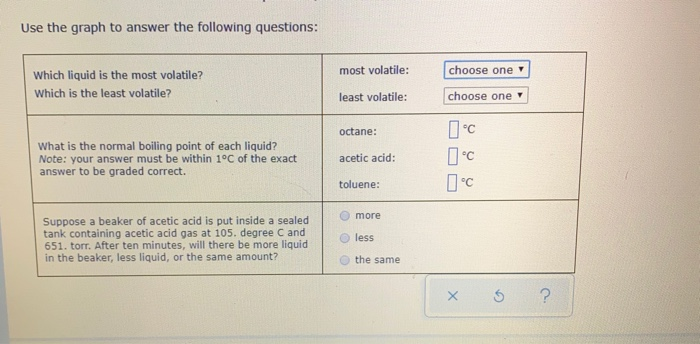Use the graph to answer the following questions: most volatile: choose one Which liquid is the most volatile? Which is the least volatile? least volatile: choose one octane: What is the normal boiling point of each liquid? Note: your answer must be within 1°C of the exact answer to be graded correct. acetic acid: toluene: more Suppose a beaker of acetic acid is put inside a sealed tank containing acetic acid gas at 105. degree C and 651. torr. After...

• ### explain how to get the center temperatures because thats where I am having most issues This graph shows how the vapor p...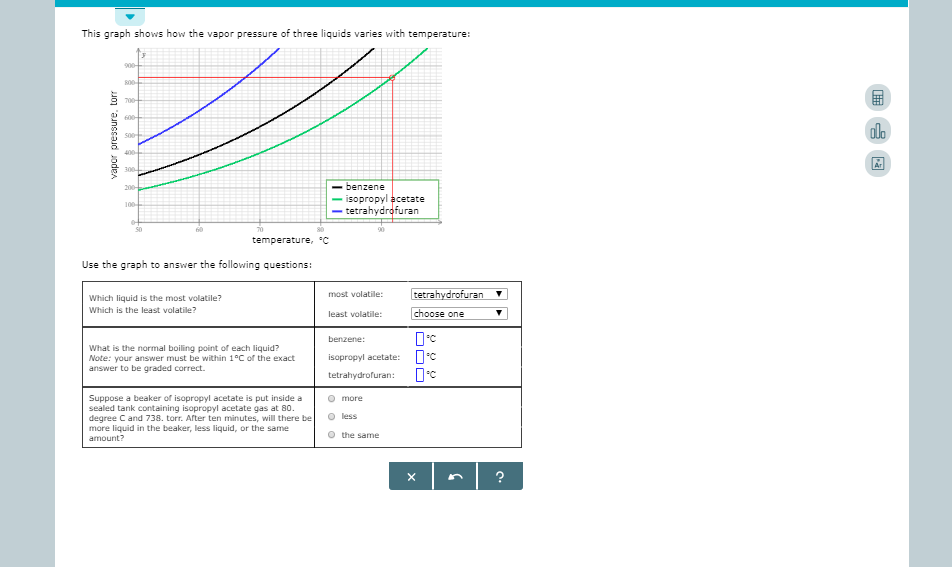explain how to get the center temperatures because thats where I am having most issues This graph shows how the vapor pressure of three liquids varies with temperature: 000- 700 600 olo s00 400 300 benzene isopropyl acetate tetrahydrofuran 700 100 60 0 80 temperature, C Use the graph to answer the following questions: tetrahydrofuran most volatile: Which liquid is the most volatile? Which is the least volatile? choose one least volatile: benzene: What is the normal boiling point of...

• ### DVANCED MATERIAL Relating vapor pressure to vaporization 100 trichloroethylene -tetrahydrofuran - ethanol 0 60 80 temperature,...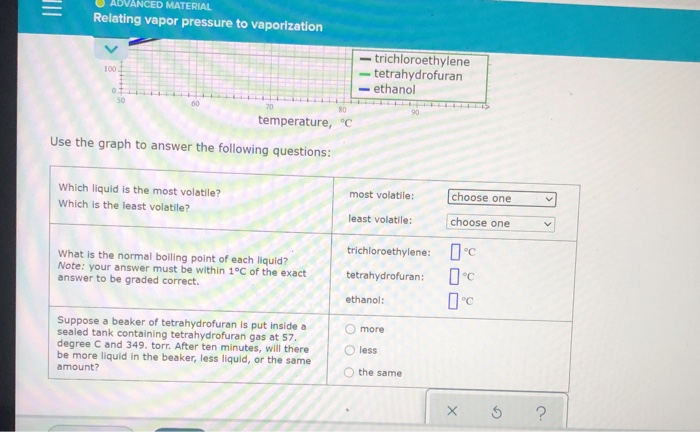DVANCED MATERIAL Relating vapor pressure to vaporization 100 trichloroethylene -tetrahydrofuran - ethanol 0 60 80 temperature, °C Use the graph to answer the following questions: Which liquid is the most volatile? Which is the least volatile? most volatile: choose one V least volatile: choose one What is the normal boiling point of each liquid? Note: your answer must be within 1°C of the exact answer to be graded correct. trichloroethylene: 1°C tetrahydrofuran: ethanol: 0°C O more Suppose a beaker of...

• ### This graph shows how the vapor pressure of three liquids varies with temperature: vapor pressure, torr...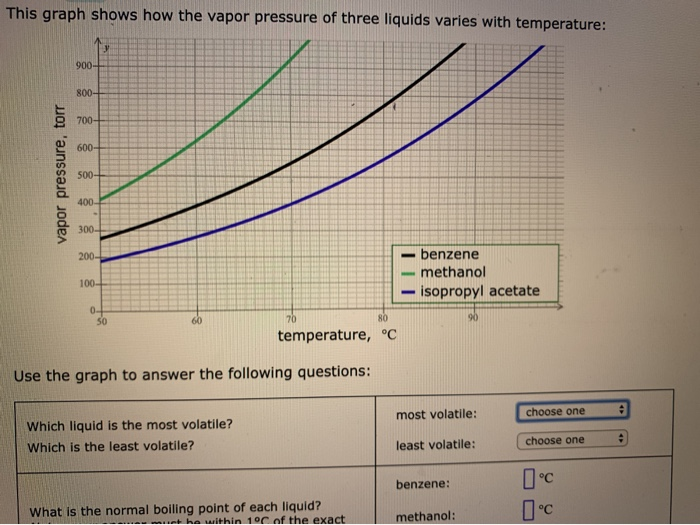This graph shows how the vapor pressure of three liquids varies with temperature: vapor pressure, torr benzene - methanol - isopropyl acetate temperature, °C Use the graph to answer the following questions: most volatile: choose one - Which liquid is the most volatile? Which is the least volatile? least volatile: choose one benzene: I°C What is the normal boiling point of each liquid? he within 10 of the exact methanol: vapor pressure - benzene -methanol isopropyl acetate temperature, °C Use...

• ### This graph shows how the vapor pressure of three liquids varies with temperature: 900 vapor pressure, torr - cycloh...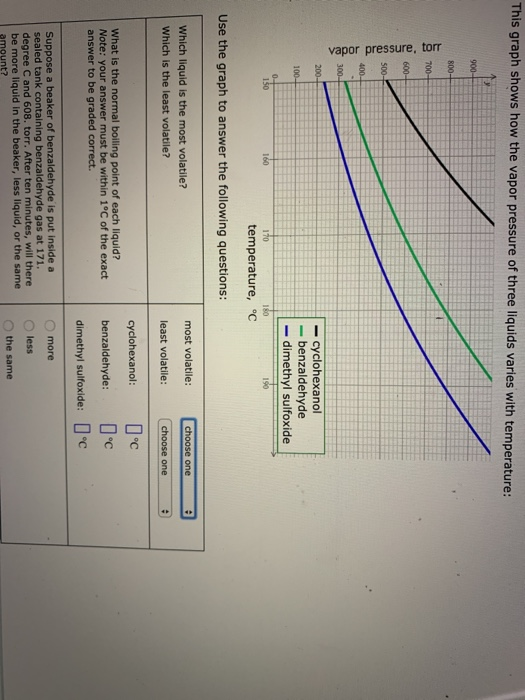This graph shows how the vapor pressure of three liquids varies with temperature: 900 vapor pressure, torr - cyclohexanol - benzaldehyde - dimethyl sulfoxide temperature, °C Use the graph to answer the following questions: most volatile: choose one - Which liquid is the most volatile? Which is the least volatile? least volatile: choose one cyclohexanol: 1 °C What is the normal boiling point of each liquid? Note: your answer must be within 1°C of the exact answer to be graded...

• ### This graph shows how the vapor pressure of three liquids varies with temperature: vapor pressure, torr...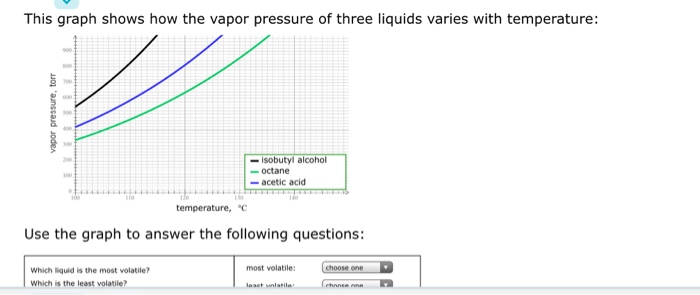This graph shows how the vapor pressure of three liquids varies with temperature: vapor pressure, torr isobutyl alcohol octane acetic acid temperature, "c Use the graph to answer the following questions: most volatile: choose one Which liquid is the most volatile? Which is the least volatile? laat wala Phancen = Relating vapor pressure to vaporization vapor pressure - isobutyl alcohol -octane -acetic acid temperature, "c Use the graph to answer the following questions: most volatile: choose one Which liquid is...

• ### This graph shows how the vapor pressure of three liquids varies with temperature: vapor pressure, torr...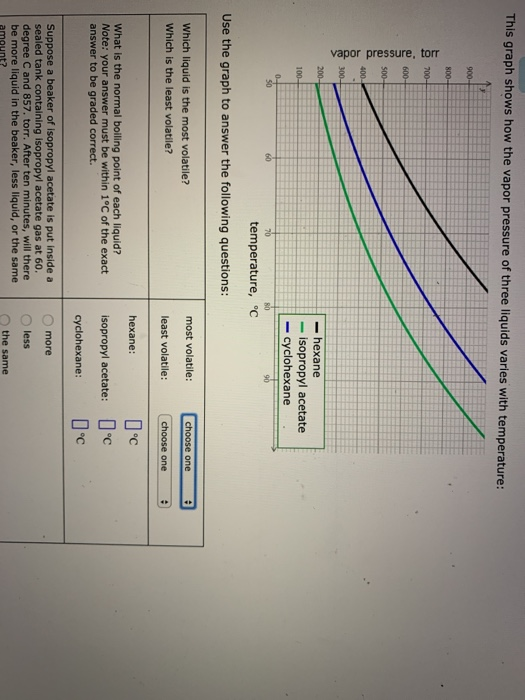This graph shows how the vapor pressure of three liquids varies with temperature: vapor pressure, torr - hexane - isopropyl acetate - cyclohexane 56 temperature, °C Use the graph to answer the following questions: most volatile: choose one Which liquid is the most volatile? Which is the least volatile? e 9 least volatile: choose one hexane: 1 °C What is the normal boiling point of each liquid? Note: your answer must be within 1°C of the exact answer to be...

• ### vapor pressure, torr This graph snows how the vapor pressure of three liquids varies with temperature:...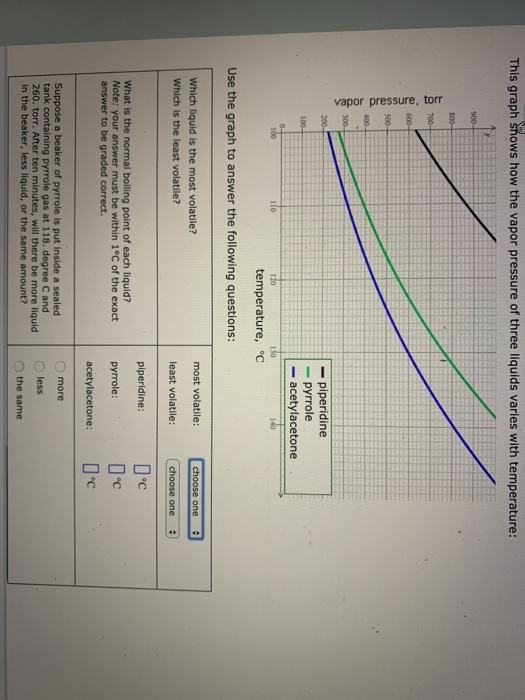vapor pressure, torr This graph snows how the vapor pressure of three liquids varies with temperature: 900- 800- 700- 600- 500- 400- 300- piperidine pyrrole acetylacetone 200- 100- 0 10 110 120 130 140 temperature, °C Use the graph to answer the following ques Which liquid is the most volatile? most volatile: choose one Which is the least volatile? least volatile: choose one piperidine: C What is the normal boiling point of each liquid? Note: your answer must be within...

• ### This graph shows how the vapor pressure of three liquids varies with temperature: 900 800- 700-...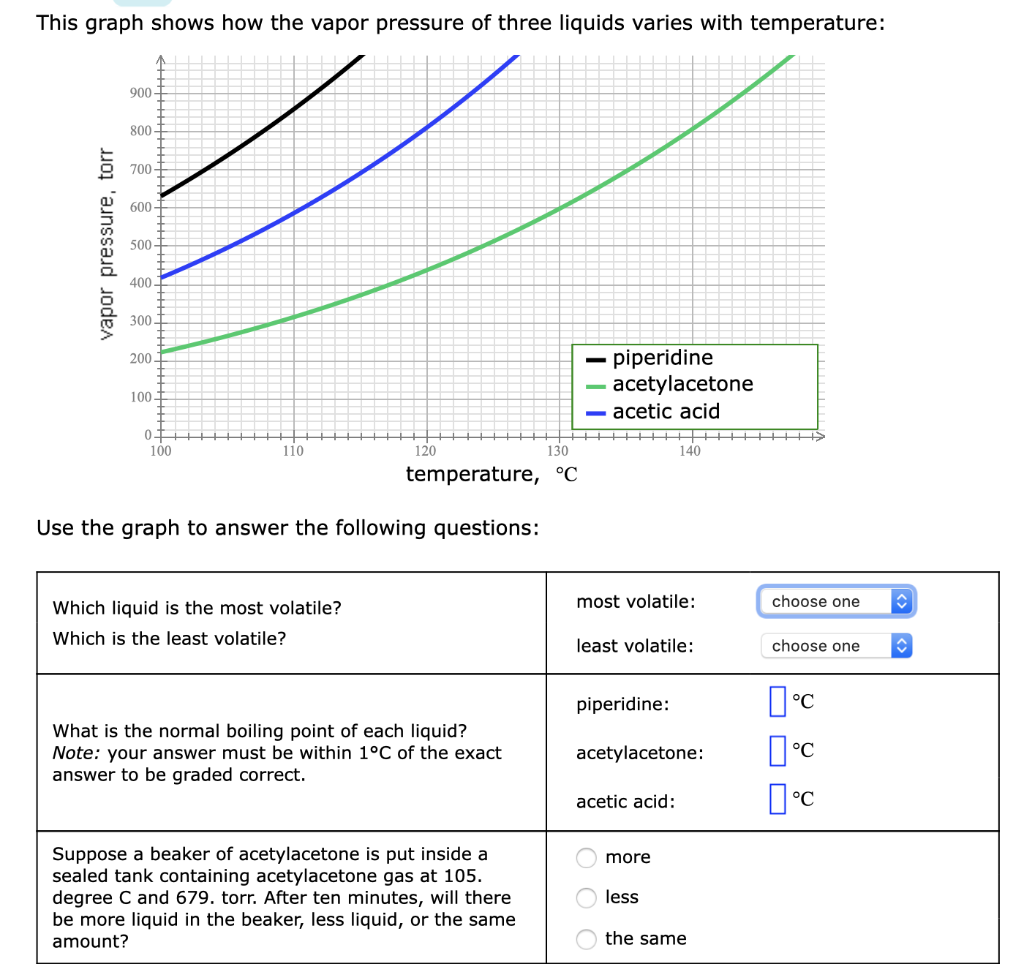This graph shows how the vapor pressure of three liquids varies with temperature: 900 800- 700- 600 vapor pressure, torr 500 400 300 200 - piperidine acetylacetone -acetic acid 100 07 100 110 120 130 140 temperature, °C Use the graph to answer the following questions: most volatile: choose one Which liquid is the most volatile? Which is the least volatile? least volatile: choose one piperidine: °c What is the normal boiling point of each liquid? Note: your answer must...